• 鱼眼相机标定方法及标定装置制造方法【专利摘要】本发明公开了一种鱼眼相机标定方法及标定装置，包括：建立所述鱼眼相机的成像模型；从不同视角采集多幅标定板图像，在所述标定板图像上获取特征点的坐标，参考所述...
鱼眼相机标定方法及标定装置制造方法【专利摘要】本发明公开了一种鱼眼相机标定方法及标定装置，包括：建立所述鱼眼相机的成像模型；从不同视角采集多幅标定板图像，在所述标定板图像上获取特征点的坐标，参考所述标定板图像的格式调整所述特征点的坐标，并根据所述标定板图像中所述特征点的预设规律，确定所述特征点的世界坐标；以RMS重投影误差为评估值，计算所述标定板图像的畸变中心偏移的初值；最小化所述RMS重投影误差，优化内参和外参。由上述内容可知，本发明提供的技术方案无需特殊的标定设备，能够适用于大多数鱼眼相机，提高了标定方法的适用性和标定结果精准度。【专利说明】鱼眼相机标定方法及标定装置【技术领域】 本发明涉及鱼眼相机【技术领域】，更为具体的说，涉及一种鱼眼相机标定方法及标 定装置。【背景技术】 近年来，由于鱼眼相机能够提供180度的视野摄像范围，在诸多领域得到了广泛 应用，例如，在机器人导航、三维建模、行车安全等领域。但是，鱼眼相机提供大角度视野摄 像范围的同时，伴随而来的是图像的鱼眼畸变，因此，鱼眼相机的应用往往需要设定鱼眼相 机的精准参数，即需要进行标定。而鱼眼相机标定结果的精度直接影响其衍生产品的精准 度，因此，标定技术得到了广泛关注。但是，采用现有标定技术受限于相机类型和特殊标定 设备的要求，适用性低；或者受限于标定计算模型，标定结果精准度差。【发明内容】 有鉴于此，本发明提供了一种鱼眼相机标定方法及标定装置，无需特殊标定设备， 适用于大部分的鱼眼相机，提高了其适用性和标定结果精准度。 为实现上述目的，本发明提供的技术方案如下： -种鱼眼相机标定方法，包括： 建立所述鱼眼相机的成像模型； 从不同视角采集多幅标定板图像，在所述标定板图像上获取特征点的坐标，参考 所述标定板图像的格式调整所述特征点的坐标，并根据所述标定板图像中所述特征点的预 设规律，确定所述特征点的世界坐标； 以RMS重投影误差为评估值，计算所述标定板图像的畸变中心偏移的初值； 最小化所述RMS重投影误差，优化内参和外参。 优选的，所述最小化所述RMS重投影误差，优化内参和外参包括： 以LM算法最小化所述RMS重投影误差，优化内参和外参。 优选的，所述建立所述鱼眼相机的成像模型包括： 获取空间中任意一景点在世界坐标系中的齐次坐标为X，在传感器平面中的投影 为U" ' = [U" '，v" ' ]T，在规范化平面中的投影为u" = [u"，v" ]T，以及，在成像平 面中的投影为U' =[U'，v' ]T，其中，所述U"与U'关系式为：【权利要求】1. 一种鱼眼相机标定方法，其特征在于，包括：  建立所述鱼眼相机的成像模型；  从不同视角采集多幅标定板图像，在所述标定板图像上获取特征点的坐标，参考所述 标定板图像的格式调整所述特征点的坐标，并根据所述标定板图像中所述特征点的预设规 律，确定所述特征点的世界坐标；  以RMS重投影误差为评估值，计算所述标定板图像的畸变中心偏移的初值；  最小化所述RMS重投影误差，优化内参和外参。2. 根据权利要求1所述的鱼眼相机标定方法，其特征在于，所述最小化所述RMS重投影 误差，优化内参和外参包括： 以LM算法最小化所述RMS重投影误差，优化内参和外参。3. 根据权利要求2所述的鱼眼相机标定方法，其特征在于，所述建立所述鱼眼相机的 成像模型包括： 获取空间中任意一景点在世界坐标系中的齐次坐标为X，在传感器平面中的投影为 U" ' = [u" '，v" ' ]τ，在规范化平面中的投影为U" = [U"，ν" ]τ，以及，在成像平面 中的投影为U' =[u'，ν' ]τ，其中，所述U"与U'关系式为：其中，Rmr = IW/IW，IW为像素宽高比，IW为显示宽高比，Rmr为存储宽高比；  所述u"'与所述u"关系式为：其中，A和T为所述内参中相机制造偏差的参数；  以及，所述鱼眼相机的成像模型为：  A*p=A*g(u"' )= λ· g(Au" +T) = λ · g(A · K(u' )+T) = P · X  其中，λ > 〇 ;p为所述鱼眼相机的视点至所述景点的向量，P为世界坐标系与相机坐 标系的透视矩阵；g为成像函数，且表示为g(u" '，V" ' ) = (U" '，V" '，f(P"')) T，f(p "')为所述鱼眼相机畸变函数，f(p " ' ) = aQ+aip " ' +a2(p " ' )2+…  aN(P " ' )N，，以及，所述鱼眼相机成像模型中的A，T，aQ，  a2, . . .，aN均为所述内参，P为所述外参。4. 根据权利要求3所述的鱼眼相机标定方法，其特征在于，所述参考所述标定板图像 的格式调整所述特征点的坐标包括： 根据所述标定板图像的格式，确定所述标定板图像的显示宽高比；根据所述标定板图 像的格式的宽高，计算所述标定板图像的存储宽高比；以及，根据公式所述Rsak = Rdak/Rpak， 计算所述标定板图像的像素宽高比；  根所述标定板图像的显示宽高比、存储宽高比、像素宽高比、所述特征点对应成像平面 的坐标和所述U"与U'关系式，计算所述特征点对应所述规范化平面的坐标。5. 根据权利要求4所述的鱼眼相机标定方法，其特征在于，所述以RMS重投影误差为评 估值，计算所述标定板图像的畸变中心偏移的初值包括： 531、 初始化所述内参A为单位阵，对所述内参T设定搜索区间范围，并对所述搜索区间 范围网格化得到多个网格点；  532、 根据所述内参A、所述网格点对应的内参T、所述特征点对应所述规范化平面的坐 标和所述u"'与所述u"关系式，计算所述特征点对应所述传感器平面的坐标，以及，计 算所述网格点对应的RMS重投影误差；  533、 以当前计算出的最小的RMS重投影误差所对应的网格点为中心，重新设定所述搜 索区间范围，并按相应比例更新网格化的所述搜索区间范围的网格大小；  534、 重复所述步骤S32至步骤S33,直至所述搜索区间范围缩小到预设精度要求，则确 定对应RMS重投影误差最小的网格点所对应的内参T为所述标定板图像的畸变中心偏移的  初值Tinit。6. 根据权利要求5所述的鱼眼相机标定方法，其特征在于，所述对所述搜索区间范围 网格化得到多个网格点为： 对所述搜索区间范围网格化为矩形网格得到m*n个网格点，且每个网格的尺寸相同，m 和η均为不小于2的整数。7. 根据权利要求6所述的鱼眼相机标定方法，其特征在于，对所述搜索区间范围网格 化为矩形网格得到7*7个网格点，且每个网格的尺寸相同。8. 根据权利要求5所述的鱼眼相机标定方法，其特征在于，所述以LM算法最小化所述 RMS重投影误差，优化内参和外参包括： 设定LM算法的优化参数为所述内参A和内参T，其中，设定所述内参A的优化初始值为 单位阵，所述内参T的优化初始值为所述畸变中心偏移的初值Tinit ;  执行所述LM算法最小化所述RMS重投影误差，得到优化后的所述内参，并参考所述鱼 眼相机的成像模型，得到优化后的外参。9. 一种鱼眼相机标定装置，其特征在于，包括： 鱼眼成像建立模块，用于建立所述鱼眼相机的成像模型；  处理模块，用于从不同视角采集多幅标定板图像，在所述标定板图像上获取特征点的 坐标，参考所述标定板图像的格式调整所述特征点的坐标，并根据所述标定板图像中所述 特征点的预设规律，确定所述特征点的世界坐标；  计算模块，用于以RMS重投影误差为评估值，计算所述标定板图像的畸变中心偏移的 初值；  优化模块，用于最小化所述RMS重投影误差，优化内参和外参。10. 根据权利要求8所述的鱼眼相机标定装置，其特征在于，所述优化模块以LM算法最 小化所述RMS重投影误差，优化内参和外参。【文档编号】G06T7/00GK104392435SQ201410627763【公开日】2015年3月4日   申请日期:2014年11月10日   优先权日:2014年11月10日【发明者】徐以强, 李庆, 闫硕, 梁艳菊, 秦瑞, 高荣, 鲁斌, 常嘉义, 陆菁   申请人:中科院微电子研究所昆山分所
展开全文• 鱼眼相机标定文献，硕士论文，鱼眼相机标定文献，硕士论文
• OpenCV相机标定包括普通相机标定和鱼眼相机标定，其中普通相机标定参考的是张正友标定法，而鱼眼相机的标定是基于等距投影模型进行标定的． 普通相机标定 张正友标定法 (1) 针孔模型内外参标定 针孔相机投影模型方程...
前言
OpenCV相机标定包括普通相机标定和鱼眼相机标定，其中普通相机标定参考的是张正友标定法，而鱼眼相机的标定是基于等距投影模型进行标定的．
普通相机标定
张正友标定法
(1) 针孔模型内外参标定
针孔相机投影模型方程:
$s\widetilde{\mathbf{m}} = \mathbf{A}\begin{bmatrix} \mathbf{R} & \mathbf{t} \end{bmatrix} \widetilde{M} \tag{1}$
式中$\widetilde{M}$表示世界坐标系下三维空间点坐标，$\widetilde{\mathbf{m}}$表示对应的投影像素坐标，$\mathbf{A}$表示相机内参，$\begin{bmatrix} \mathbf{R} & \mathbf{t} \end{bmatrix}$表示相机外参(世界坐标系与相机坐标系之间的坐标变换关系)，$s$表示任意尺度因子．
对公式(1)进行展开，可得下式:
$s\begin{bmatrix} u \\ v\\ 1\end{bmatrix} = \mathbf{A}\begin{bmatrix} \mathbf{r}_{1} & \mathbf{r}_{2} & \mathbf{r}_{3} & \mathbf{t} \end{bmatrix} \begin{bmatrix} X \\ Y \\ Z\\ 1\end{bmatrix} \tag{2}$
式中$(\mathbf{r}_{1}, \mathbf{r}_{2}, \mathbf{r}_{3})$表示旋转矩阵$\mathbf{R}$的三个列向量．将世界坐标系定义在一块标定板上(z轴垂直与标定板平面)，则标定板上任意三维空间点坐标可表示为$(X, Y, 0)$，基于此定义，可将公式(2)简化为:
$s\begin{bmatrix} u \\ v\\ 1\end{bmatrix} = \mathbf{A}\begin{bmatrix} \mathbf{r}_{1} & \mathbf{r}_{2} & \mathbf{t} \end{bmatrix} \begin{bmatrix} X \\ Y \\ 1\end{bmatrix} = \mathbf{H}\begin{bmatrix} X \\ Y \\ 1\end{bmatrix} \tag{3}$
设$\mathbf{H}=\begin{bmatrix} \mathbf{h}_{1} & \mathbf{h}_{2} & \mathbf{h}_{3} \end{bmatrix}$，则有:
$\begin{bmatrix} \mathbf{h}_{1} & \mathbf{h}_{2} & \mathbf{h}_{3} \end{bmatrix} = \lambda \mathbf{A}\begin{bmatrix} \mathbf{r}_{1} & \mathbf{r}_{2} & \mathbf{t} \end{bmatrix} \tag{4}$
将$\mathbf{A}$移到公式(4)左边，有:
$\begin{bmatrix} \mathbf{A}^{-1}\mathbf{h}_{1} & \mathbf{A}^{-1}\mathbf{h}_{2} & \mathbf{A}^{-1}\mathbf{h}_{3} \end{bmatrix} = \lambda\begin{bmatrix} \mathbf{r}_{1} & \mathbf{r}_{2} & \mathbf{t} \end{bmatrix} \tag{4}$
由于$(\mathbf{r}_{1},\mathbf{r}_{2})$是旋转矩阵$\mathbf{R}$的两个列向量，并且旋转矩阵是正定的，所以$(\mathbf{r}_{1},\mathbf{r}_{2})$是标准正交向量组，即满足下式:
$\left\{\begin{matrix} \mathbf{r}_{1}\cdot \mathbf{r}_{2} = 0 = (\mathbf{A}^{-1}\mathbf{h}_{1})^{T}(\mathbf{A}^{-1}\mathbf{h}_{2}) = \mathbf{h}_{1}^{T}\mathbf{A}^{-T}\mathbf{A}^{-1}\mathbf{h}_{2}\\ \mathbf{r}_{1}\cdot \mathbf{r}_{1} = 1 = (\mathbf{A}^{-1}\mathbf{h}_{1})^{T}(\mathbf{A}^{-1}\mathbf{h}_{1}) = \mathbf{h}_{1}^{T}\mathbf{A}^{-T}\mathbf{A}^{-1}\mathbf{h}_{1} \\ \mathbf{r}_{2}\cdot \mathbf{r}_{2} = 1 = (\mathbf{A}^{-1}\mathbf{h}_{2})^{T}(\mathbf{A}^{-1}\mathbf{h}_{2}) = \mathbf{h}_{2}^{T}\mathbf{A}^{-T}\mathbf{A}^{-1}\mathbf{h}_{2} \end{matrix}\right. \tag{5}$
简化公式(5)可得:
$\left\{\begin{matrix} \mathbf{h}_{1}^{T}\mathbf{A}^{-T}\mathbf{A}^{-1}\mathbf{h}_{2} = 0\\ \mathbf{h}_{1}^{T}\mathbf{A}^{-T}\mathbf{A}^{-1}\mathbf{h}_{1} = \mathbf{h}_{2}^{T}\mathbf{A}^{-T}\mathbf{A}^{-1}\mathbf{h}_{2} \end{matrix}\right. \tag{6}$
将公式(6)中的 $\mathbf{A}^{-T}\mathbf{A}^{-1}$ 看成一个整体，可以将其转换成齐次方程组$\mathbf{A_{i}x} = \mathbf{0}$的形式，由于 $\mathbf{A}^{-T}\mathbf{A}^{-1}$ 的自由度为6，因此至少需要三组匹配点构成至少6个方程来求解齐次方程组中的$\mathbf{x}$，对于齐次方程组的求解可利用svd方法，此处求解的详细过程是简单的数值分析的知识(略)．通过多组匹配点求解出相机内参矩阵$\mathbf{A}$之后，可通过下式计算出外参矩阵:
\begin{aligned} \mathbf{r}_{1} &= \lambda\mathbf{A}^{-1}\mathbf{h}_{1} \\ \mathbf{r}_{2} &= \lambda\mathbf{A}^{-1}\mathbf{h}_{2} \\ \mathbf{r}_{3} &= \mathbf{r}_{1} \times \mathbf{r}_{2} \\ \mathbf{t} &= \lambda\mathbf{A}^{-1}\mathbf{h}_{3} \end{aligned} \tag{7}
(2) 引入畸变模型
张正友标定法中只考虑了径向畸变，其畸变方程如下:
$\begin{bmatrix} (u - u_{0})(x^{2} + y^{2}) & (u - u_{0})(x^{2} + y^{2})^{2} \\ (v - v_{0})(x^{2} + y^{2}) & (v - v_{0})(x^{2} + y^{2})^{2} \end{bmatrix} \begin{bmatrix} k_{1} \\ k_{2} \end{bmatrix}= \begin{bmatrix} \check{u} - u \\ \check{v} - v \end{bmatrix} \tag{8}$
式中 $(u,v)$ 表示理想的通过针孔模型获得的投影像素坐标， $(\check{u},\check{v})$ 表示实际的投影像素坐标．通过式(8)可以看出一对配对点可以构建两个方程，因此通过多对匹配点构建超定方程，然后再通过SVD分解或者QR分解来求解构建的超定方程，从而可以获得畸变系数 $(k_{1}, k_{2})$．在获得畸变系数之后可以对先前计算获得的内外参数进行精细化，其方法是通过构建重投影残差方程，然后通过优化残差获得精细化的内外参数及畸变系数，残差方程构建如下:
$\Sigma_{i=1}^{n}\Sigma_{j=1}^{m}\left\| \mathbf{m}_{ij} - \check{\mathbf{m}}(\mathbf{A},k_{1},k_{2},\mathbf{R}_{i}, \mathbf{t}_{i}, \mathbf{M}_{j}) \right\|^{2} \tag{9}$
式中 $\mathbf{m}_{ij}$ 表示世界坐标系下的点 $\mathbf{M}_{j}$ 在第$j$张图像上的投影点，$\check{\mathbf{m}}$ 表示通过内外餐及畸变系数计算出的投影点坐标．上述方程组是非线性方程组，可通过高斯牛顿或LM方法进行迭代求解，至此实现了相机的所有参数的标记: 内参、外参、畸变系数．
(3) 标定流程

打印标定板并粘贴到平面上
在相机镜头前移动标定板获取几张照片
检测图像中的特征点(标定板角点)
使用公式(6)构建的方程组计算相机内参和外参
使用公式(8)计算相机畸变
使用公式(9)对相机内外参和畸变系数进行进一步精细化．

OpenCV标定算法
OpenCV对于普通相机的标定方法基本上与张正友标定法一致，只是其考虑了相机的径向畸变和桶形畸变，分别如下：
径向畸变:桶形畸变:因此其畸变参数为$(k_{1}, k_{2}, p_{1}, p_{2}, p_{3})$. 其余参数和流程与张正友标定一致．
鱼眼相机标定
OpenCV中将鱼眼相机区分开进行标定，原因是等距投影模型更能真实的刻画鱼眼相机的投影过程，其投影方程如下:上面公式中$\mathbf{X}$表示世界坐标系下的三维点，$(\mathbf{R}, \mathbf{t})$表示相机外参， $\mathbf{X}_{c}$表示相机坐标系下的三维点，$(u, v)$为投影的像素坐标．该投影模型与普通相机的投影模型相比反而更简单，其标定的参数包括相机内参$(f_{x}, f_{y}, c_{x}, c_{y})$，外参$(\mathbf{R}, \mathbf{t})$，以及畸变系数$(k1, k2, k3, k4)$．标定的过程与普通相机的标定过程基本一致，只是投影模型不一样而已，最后也是构建重投影残差方程，然后优化方程获取相机的内外参及畸变系数．
标定程序
https://github.com/chennuo0125-HIT/opencv_demo/tree/master/calibrate
标定结果展开全文• 开始标定double fisheye::calibrate(InputArrayOfArrays objectPoints, InputArrayOfArrays imagePoints,const Size& image_size, InputOutputArray K, InputOutputArray D,OutputArrayOfArrays rvecs, ...
开始标定double fisheye::calibrate(InputArrayOfArrays objectPoints, InputArrayOfArrays imagePoints, const Size& image_size, InputOutputArray K, InputOutputArray D, OutputArrayOfArrays rvecs, OutputArrayOfArrays tvecs, int flags=0, TermCriteria criteria=TermCriteria(TermCriteria::COUNT + TermCriteria:: EPS, 100, DBL_EPSILON))注意：K，D 分别表示内参矩阵和畸变系数向量，在定义时要定义为double型，这里推荐使用Matx33d和Vec4d数据类型，更为方便简单。objectPoints，imagePoints可以是float型，也可以是double型，但是再stereorectify中需要时double型。flags的可选项有很多，其中需要注意的是必须要指定CALIB_FIX_SKEW，代表求解时假设内参中fx=fy.4.评定误差(可选项)for (int i = 0; i != image_count; i++){cout << "Frame #" << i + 1 << "..." << endl;string image_Name;stringstream stream;stream << (i + startNum);stream >> image_Name;image_Name = path_ChessboardImage + image_Name + ".jpg";cv::Mat image = imread(image_Name);Mat image_gray;cvtColor(image, image_gray, CV_RGB2GRAY);vector corners;bool patternFound = findChessboardCorners(image_gray, board_size, corners,CALIB_CB_ADAPTIVE_THRESH + CALIB_CB_NORMALIZE_IMAGE + CALIB_CB_FAST_CHECK);if (!patternFound || fullcornersNum != corners.size()){cout << "can not find chessboard corners!\n";continue;}else{cornerSubPix(image_gray, corners, Size(11, 11), Size(-1, -1), TermCriteria(CV_TERMCRIT_EPS + CV_TERMCRIT_ITER, 30, 0.1));count = count + corners.size();corners_Seq.push_back(corners);successImageNum = successImageNum + 1;image_Seq.push_back(image);}}/************************************************************************摄像机定标*************************************************************************/vector>  object_Points;        /****  保存定标板上角点的三维坐标   ****/Mat image_points = Mat(1, count, CV_32FC2, Scalar::all(0));  /*****   保存提取的所有角点   *****/vector  point_counts;/* 初始化定标板上角点的三维坐标 */for (int t = 0; t{vector tempPointSet;for (int i = 0; i{for (int j = 0; j{/* 假设定标板放在世界坐标系中z=0的平面上 */Point3f tempPoint;tempPoint.x = i*square_size.width;tempPoint.y = j*square_size.height;tempPoint.z = 0;tempPointSet.push_back(tempPoint);}}object_Points.push_back(tempPointSet);}for (int i = 0; i< successImageNum; i++){point_counts.push_back(board_size.width*board_size.height);}/* 开始定标 */Size image_size = image_Seq.size();cv::Matx33d intrinsic_matrix;    /*****    摄像机内参数矩阵    ****/cv::Vec4d distortion_coeffs;     /* 摄像机的4个畸变系数：k1,k2,k3,k4*/std::vector<:vec3d> rotation_vectors;                           /* 每幅图像的旋转向量 */std::vector<:vec3d> translation_vectors;                        /* 每幅图像的平移向量 */int flags = 0;flags |= cv::fisheye::CALIB_RECOMPUTE_EXTRINSIC;flags |= cv::fisheye::CALIB_CHECK_COND;flags |= cv::fisheye::CALIB_FIX_SKEW;fisheye::calibrate(object_Points, corners_Seq, image_size, intrinsic_matrix, distortion_coeffs, rotation_vectors, translation_vectors, flags, cv::TermCriteria(3, 20, 1e-6));标定结果：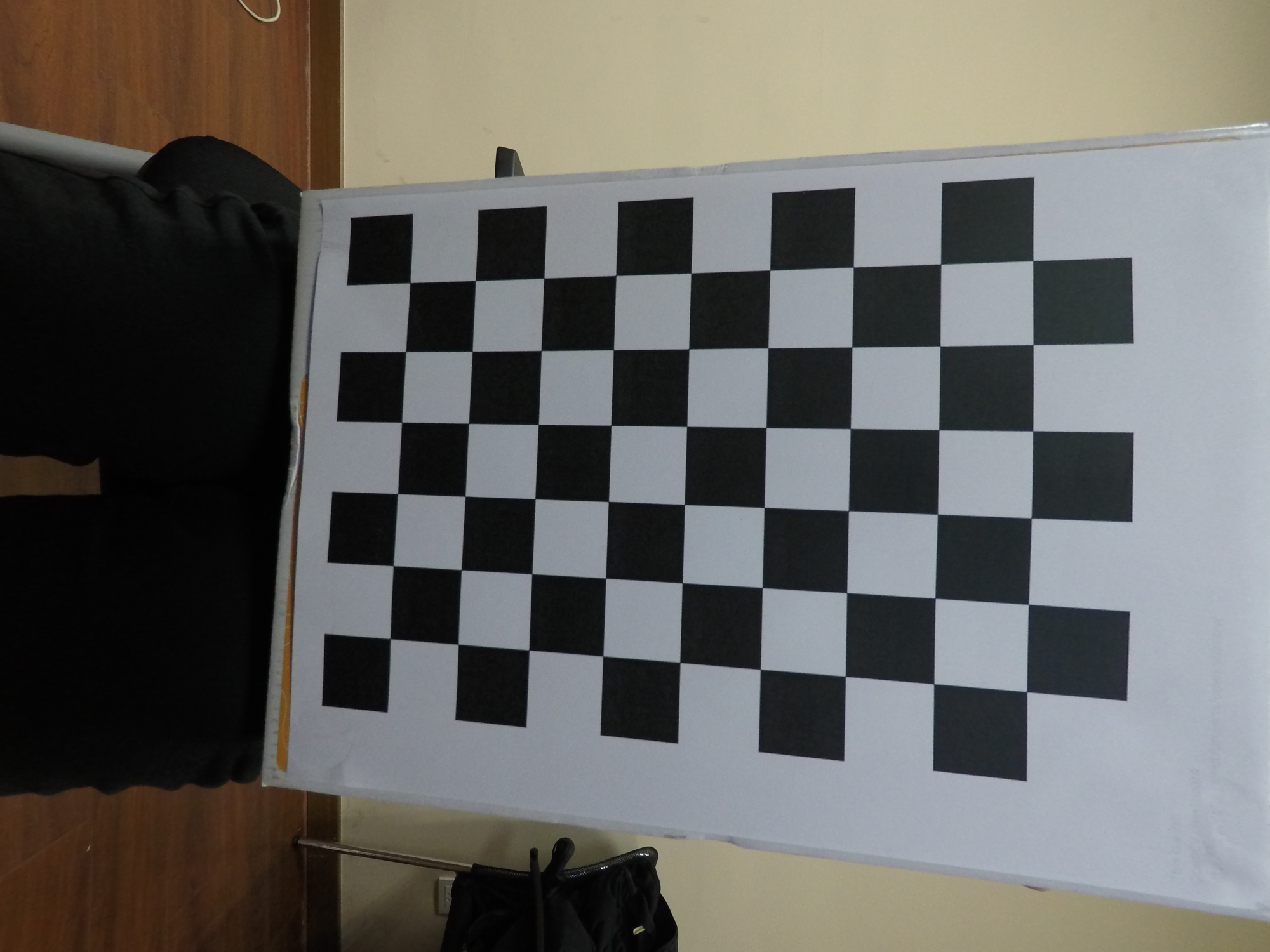展开全文• ，与普通镜头的内参一样，但畸变参数不同，为 ，含义如下： 设(X,Y,Z)为一个三维坐标点，投影在图像上的二维坐标为(u,v)，如果不考虑畸变，投影关系如下： R和t分别代表相机外参中的旋转矩阵和平移向量。 标定流程 ...
【原文转自】：http://blog.csdn.net/qq_15947787/article/details/51441031Kannala J, Brandt S S. A generic camera model and calibration method for conventional, wide-angle, and fish-eye lenses[J]. Pattern Analysis and Machine Intelligence, IEEE Transactions on, 2006, 28(8): 1335-1340.文献地址：http://download.csdn.net/detail/qq_15947787/9583006opencv也是参考matlab的Calib_gui_fisheye处理，但是不清楚为什么，Calib_gui_fisheye不能准确的提取角点鱼眼镜头模型鱼眼镜头的内参模型可以表示为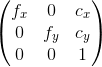，与普通镜头的内参一样，但畸变参数不同，为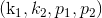，含义如下：设(X,Y,Z)为一个三维坐标点，投影在图像上的二维坐标为(u,v)，如果不考虑畸变，投影关系如下：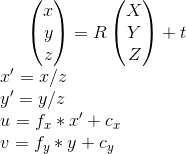R和t分别代表相机外参中的旋转矩阵和平移向量。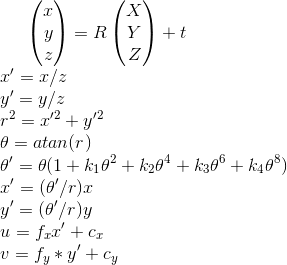标定流程首先调用OpenCV的FindChessboardCorners()来寻找图像上的标定板的角点，再根据标定板的尺寸指定这些角点对应的三维点的三维坐标，再调用fisheye::calibrate()来进行标定，利用标定结果中的内参和畸变参数调用fisheye::undistortImage()对图像做去畸变操作。最后调用一张待测试的畸变图片利用标定结果进行畸变校正。后续，两个代码下载链接：2016/12/2 20：00在做双目标定及空间点计算时，突然看到stereoRectify有一个alpha参数，可以调节校正图的大小，而initUndistortRectifyMap中的size是校正图的大小，并不是原图大小，这样应该就好办了吧。http://download.csdn.net/detail/qq_15947787/9700379
展开全文• 鱼眼相机标定校正+批量加载多张标定图像+批量处理多张测试图像+角点坐标输出+相机参数输出
• 鱼眼相机标定 前段时间曾经做过一段时间的摄像头标定，这里对以前做的事情做一个总结。这里介绍一下鱼眼相机的标定吧，也是相机标定的第二部分，主要还是代码解析和一些细节说明，为了让自己更好的理解相机标定，...机器视觉
• 鱼眼相机标定，有时候也可以用普通相机的标定方法对其进行标定，但是却不能保证去畸变后的效果是最好的。因此对于Gopro等鱼眼镜头拍摄出来的图像去畸变，最好的方法就是采用鱼眼相机标定方法进行标定。 鱼眼相机
• ## 鱼眼相机标定以及OpenCV实现

万次阅读 热门讨论 2016-01-07 12:22:18
鱼眼相机标定，有时候也可以用普通相机的标定方法对其进行标定，但是却不能保证去畸变后的效果是最好的。因此对于Gopro等鱼眼镜头拍摄出来的图像去畸变，最好的方法就是采用鱼眼相机标定方法进行标定。畸变模型
• 鱼眼相机标定与矫正FECC1.0（FishEye Camera Calibration and correction） 下载后请阅读ReadMe文档，有问题可以在评论区评价，我有时间会根据评价发布新版本 程序说明 1. 将待校正的拍摄棋盘格的图像放置于Len...
• 一、简介 本文主要介绍鱼眼镜头成像和内参标定技术，结合实际应用场景，解决不同领域使用鱼眼镜头进行内参标定的应用难题。 什么是鱼眼镜头？鱼眼镜头是一种焦距为16mm或更短的并且视角接近或等于180°的镜头。因其...
• 利用opencv图像算法库实现对鱼眼相机内外参的标定，并利用标定结果对畸变图像进行校正。opencv
• Camera Calibration是计算Camera的...你同样可以在在一个鱼眼相机中获得未失真的图像。 鱼眼相机可以被用于里程计（odometry）以及vslam（simultaneous localization and mapping）。其他应用中，有包括监控系统...
• 基于2D模板与3D包围式标定块的鱼眼相机标定,主要是介绍如何定标鱼眼相机的畸变
• 前言在介绍其他相机模型之前，可以先看一下kalibr支持标定相机模型（kalibr可以标定相机模型），这里的相机模型一共有4种，针孔相机模型，全景相机模型，Double sphere相机模型还有EUCM。其中EUCM已经在前一篇...
• 本文是MATLAB鱼眼相机标定过程，建立在学习普通相机的标定过程之上。转载请注明出处。请高手指教结果出现更加畸形的原因。 普通相机的标定引用自：http://blog.csdn.net/jameshater/article/details/53172333 好多...MATLAB
• 这是用opencv做的相机标定，去畸变和逆投影。并且工程里还含有测试图像，是一个180度的鱼眼相机拍摄的棋盘格，能应对各种广角和鱼眼相机的标定。项目工程是在平台vs2015，在其他平台请自己修改一下工程属性，请添加...
• unified omnidirectional camera model前一篇EUCM其实是该相机模型的一个扩展，该相机模型有5个参数 ，它刚开始是针对于大FOV的鱼眼相机提出的，并且有两个优点：(1)它能精确的模拟各种图像设备和畸变的几何图像...
• Camera Calibration是计算Camera的...你同样可以在在一个鱼眼相机中获得未失真的图像。鱼眼相机可以被用于里程计（odometry）以及vslam（simultaneous localization and mapping）。其他应用中，有包括监控系统（...
• 手头有一个双目鱼眼相机，虽然厂家SDK里提供了相应的参数，但中间发现一点小问题，索性自己重新标定一下。单个摄像头的鱼眼标定在MATLAB工具箱中就有，但双目标定却是没有。找了一下OpenCV中（应该是3.0以后版本的）...OpenCV 双目标定
• undistorted_img) img2 = cv2.imread(images) cv2.imshow("none undistorted", img2) cv2.waitKey(0) cv2.destroyAllWindows() 使用时修改CHECKERBOARD参数即可，改为自己使用的标定板的内部角点数
• undistorted_img) img2 = cv2.imread(images) cv2.imshow("none undistorted", img2) cv2.waitKey(0) cv2.destroyAllWindows() 使用时修改CHECKERBOARD参数即可，改为自己使用的标定板的内部角点数
• 参照opencv及网上资料基于opencv3.0编写，标定结果与matlab2015一致，欢迎交流！...